# Stationary Point of Variation of Action

Trying2Learn
TL;DR Summary
How do we know it is a minumum
The action is the time integral of the Lagrangian - check.

We take the variation of it, and set it to zero - check

But how do we know it is a minimum and not a maximum?

(Don't we formally have to deal with the second variation to be positive?)

Or am I confused? Is it really just important to know it is stationary, and not necessarily a minimum or maximum (and, if so, why?)

Homework Helper
Gold Member
2022 Award
If we are talking about a classical Lagrangian then typically there can be no local maxima for physical reasons. If you take an example, can you see why?

Trying2Learn
I am sorry, I cannot see why.
In fact, if you look at my second post this morning, you will see my stupidity is even greater

Homework Helper
Gold Member
2022 Award
I am sorry, I cannot see why.
What about the simplest example of a free particle with no PE function?

Trying2Learn
What about the simplest example of a free particle with no PE function?
OK, I can see that in the case of a mechanical system with NO PE, and with KE = to the velocity squared, there will be no local maxima.

But that just postpones the issue for me. For in any calculus of variations book, they search for an extrema.

And that now makes me think I do not understand varying the Action at all.

I thought is was about MINIMIZING the action. Now I wonder if it is ony about finding and extrema.

And I wonder why the books switch from calling it an extrema and the switching to minimal, without an explanation.

I am confused.

(even more so with the other question I posted -- and I am hoping you can fix me there, too.)

Homework Helper
Gold Member
2022 Award
In general, we are looking for extrema of the action. Whether that's a maximum or a minimum depends on the system. For a free classical particle we are minimising the action (straight line at constant velocity), but for a relativistic free particle we are maximising the action/proper time (again a straight line at constant velocity).

You can generally work out whether it's a maximum or minimum (I don't know about saddle points off the top of my head) but the Euler-Lagrange equations work the same in both cases.

It's not supposed to be a big issue!

Trying2Learn
OK, so if I understand correctly, then the expression "Principle of Least Action" is absurd.

It SHOULD be called: the "Principle of Extreme action" since we are not, in general, concerned with whether it is a local minimum or maximum (yes in a mechanical problem, it is a minimum, but that is not the case for all problems as you point out).

So when using it in mechanics, we only try to find the stationary values of the Action, not necessarily the minimum.

Is that correct?

•Dale
Homework Helper
Gold Member
2022 Award
It's not absurd. It started out for historical reasons as least action and in more modern terms is seen to be extreme action. That level of linguistic anomaly shouldn't be a barrier to understanding!

Mentor
It SHOULD be called: the "Principle of Extreme action" since we are not, in general, concerned with whether it is a local minimum or maximum
Yes. We are just extremising the action.

Trying2Learn
For PeroK

I am unable to post the Matlab code (so you cannot see the plot of the diff. of KE and PE and the total Action)

However, here is the core of the numerical part (about which, my question is at the end)

%Declare symbolic variables (explained as I use them)
syms x x_dot t Pe Ke L eta

%Set mass and gravity (could be any number)
m = 1;
g = 9.8;

%Initial Height (could be any number)
H = 10;

%Initial Velocity (could be any number)
V = 2;

%Set end time and start time: you can vary these and play
t0 = 0;
t1 = 3;

%Set a time range for integrating
range = [t0,t1];

%I now have TWO epsilons: one to vary the position and one to vary the
%velocity. Right now, they are both 0, but you can increase them and see
%the impact on the Action
epsilonp = 0.0;
epsilonv = 0.0;

% The following varying function is 0 at the endpoints: eta.
eta(t) = t*t*(t-range(2));

%Compute the velocity of the falling object + some variation
x_dot(t) = -g*t + V + epsilonv * eta(t);

%Compute the position + some variation
x(t) = -0.5 * g * t*t + V*t + H + epsilonp * eta(t);

%Potential Energy
Pe(t) = m*g*x(t);

%Kinetic Energy
Ke(t) = 0.5*m*x_dot(t)^2;

%Lagrangian
L(t) = Ke(t) - Pe(t);

%Integrate over the time start and stop
A(t) = int(L(t),t0, t1);

%THE NEXT PART IS ONLY PLOTTING. YOU CAN SKIP THIS AND GO TO LINE 79 to find
%the integral of each action

Now my question is this:
Am I really testing the Action Extremum properly?
I mean, I use the same eta function, but I have different epsilons.
It still strikes me as odd that I have TWO different epsilons, but one variation

Homework Helper
Gold Member
2022 Award
I don't know anything about Matlab, so someone else will have to take a look at this.

Homework Helper
Gold Member
2022 Award
The technique used for deriving the E-L equations involves something of a trick. We imagine we have a path through the N-dimensional space of coordinates and first derivatives. Now, imagine any other path that begins and ends at the same points. The difference in those paths is a third path, which we'll call the variation. Since the variation is zero at the end points, wee may multiply that path by any positive real number ##\epsilon## and we still have a valid variation (still matches the endpoints).

The trick is to realize if the original path is a minimum, then it is a minimum for the set of paths defined by our particular variation and the parameter ##\epsilon##. This allows us to transform the problem that involves considering every possible path to one where we consider paths parameterised in this way and hence a standard single-variable calculus problem: it must be a minimum when ##\epsilon = 0##.

If you vary every coordinate separately, then in principle you should still be able to generate the E-L equations, but it will probably get messier than using the parameterisation trick above.

Trying2Learn
The technique used for deriving the E-L equations involves something of a trick. We imagine we have a path through the N-dimensional space of coordinates and first derivatives. Now, imagine any other path that begins and ends at the same points. The difference in those paths is a third path, which we'll call the variation. Since the variation is zero at the end points, wee may multiply that path by any positive real number ##\epsilon## and we still have a valid variation (still matches the endpoints).

The trick is to realize if the original path is a minimum, then it is a minimum for the set of paths defined by our particular variation and the parameter ##\epsilon##. This allows us to transform the problem that involves considering every possible path to one where we consider paths parameterised in this way and hence a standard single-variable calculus problem: it must be a minimum when ##\epsilon = 0##.

If you vary every coordinate separately, then in principle you should still be able to generate the E-L equations, but it will probably get messier than using the parameterisation trick above.

If I were to vary each coordinate separately (one epsilon for the velocity and another for the position), and set that to zero, would I then not have TWO expressions, instead of the ONE E-L?

Essentially, what is the FORM of the variation?

Because I am thinking this:

Partial of Action = ∂/∂ε1 +∂/∂ε2

And then I get TWO terms for the EL, not one.

I am very dense. Sorry

Homework Helper
Gold Member
2022 Award

If I were to vary each coordinate separately (one epsilon for the velocity and another for the position), and set that to zero, would I then not have TWO expressions, instead of the ONE E-L?

Essentially, what is the FORM of the variation?

Because I am thinking this:

Partial of Action = ∂/∂ε1 +∂/∂ε2

And then I get TWO terms for the EL, not one.

I am very dense. Sorry
All those equations must be true simultaneously. But, also, those equations by themselves may not be sufficient to generate E-L. This is not something I've ever looked at.

Trying2Learn
All those equations must be true simultaneously. But, also, those equations by themselves may not be sufficient to generate E-L. This is not something I've ever looked at.
OK, then can you tell me in a simple way, HOW it is possible to vary the position and the velocity INDEPENDENTLY, yet in such a way that the variations are derived from each other?

Because right now, I suspect the whole game as fishy, because if we ARE using
• Variation of position: epsilon * eta(t)
• Variation of velocity: epsilon * d(eta(t))/dt

Then, well, those don't look like independent variations to me.

Last edited:
Homework Helper
Gold Member
2022 Award
OK, then can you tell me in a simple way, why it is possible to create a variation of the position and the velocity in such a way that the variations are derived from each other?

Because right now, I suspect the whole game as fishy, because if we ARE using
• Variation of position: epsilon * eta(t)
• Variation of velocity: epsilon * d(eta(t))/dt

Then, well, those don't look like independent variations to me.

That's well spotted! Effectively, we have constrained ourselves to looking at variations of the path that make "physical sense" - in that one respect. It's true that this is a subset of all possible variations, but even so, if the action is a minimum, it must be a minimum for this subset as well.

It turns out that considering this reduced set of variations is sufficient to generate the E-L equations. And, we can show that Newton's Laws are equivalent to the E_L equations. That's the objective.

I'm not sure exactly what happens if you consider all possible variations - I haven't ever looked into that.

Gold Member
You ask: "How we know it is a minimum?"

Indeed it isn't necessary to know whether you are dealing with a minimum or a maximum.

The true criterium is that the derivative of Hamilton's action is zero.

Mathematically, when you have a function with a point where derivative is zero that point will be an extremum, but in the case of Hamilton's stationary action that extremum is incidental.

To see why it is immaterial I will discuss calculus of variations.
(The following is mostly copied from a discussion that I submitted on physics.stackexchange)

In differential calculus the unit of operation is a pair of points, the line through those two points is tangent to the curve you are looking to solve for.

In calculus of variations the unit of operation is a triplet of points.

Diagram 1 is an animated GIF that represents that unit of operation.
(The animated GIF is composited from screenshots of an interactive diagram.)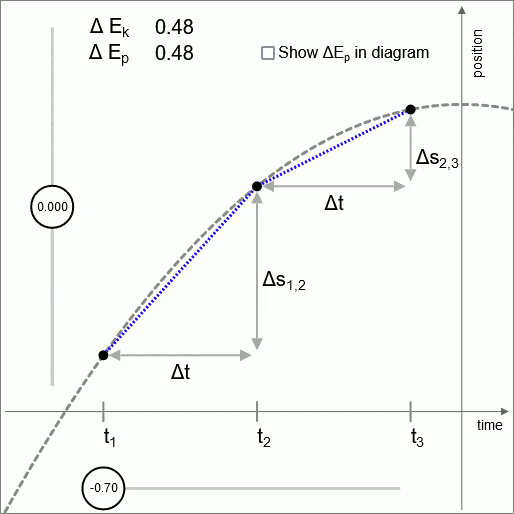Diagram 1 - Unit of operation

In diagram 1 the intermittent line is a parabola. This parabola represents height as a function of time of an object that has been thrown upwards. A uniform force is acting in downward direction. To obtain the simplest possible parabola the value of the (uniform) acceleration is set at 2 ##m/s^2##

The outer points (##t_1## and ##t_3##) are treated as fixed; variation is executed by varying the position coordinate of the middle point.

In the diagram two adjacent time intervals are evaluated; ##t_{1,2}## and ##t_{2,3}##

The sweet spot is the point where the change of velocity is such that the difference in kinetic energy matches the difference in potential energy.

(In this diagram a uniform acceleration is used, which means that in this diagram the potential increases linear with height, hence ##\Delta E_p## is in this diagram constant. In the general case the force changes as a function of the position coordinate. Then the potential is not linear, and the value of ##\Delta E_p## changes as a function of the position coordinate.)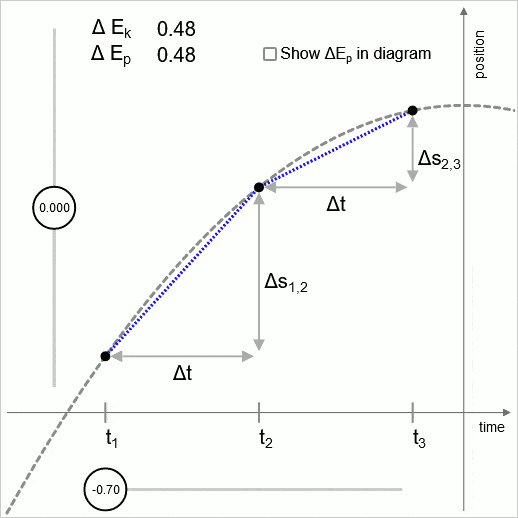Diagram 2 - valid at any scale

Diagram 2 illustrates that the logic of this unit of operation is valid at any scale, down to the limit of infinitisimal time intervals.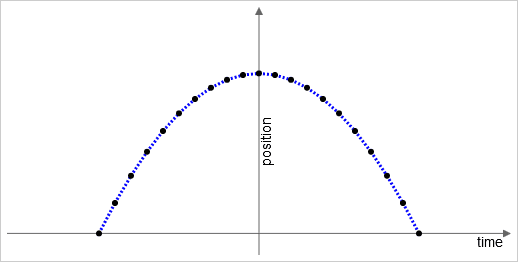Diagram 3 - Concatenation

Diagram 3 illustrates concatenation of the unit of operation over the entire domain of the function you are looking to solve for.

The analogy with the unit of operation of differential calculus: you concatenate the unit of operation over the domain of the function you are looking to solve for, and then you take the limit of infinitisimally small intervals.

As an example of a source where this idea of concatenation of the unit of operation is discussed: the book 'Calculus of variations' by Gelfand and Fomin. Gelfand and Fomin demonstrate that the Euler-Lagrange equation can be derived using the functional derivative concept.

This representation as a concatenation is without loss of generality.
For instance, in the case of Hooke's law the resulting trajectory is the sine function, which when expanded as a Taylor series has an infinite number of terms. The concatenation accommodates that.

the diagrams 1, 2, and 3 illustrate that the logic can be stated such that it applies at infinitisimal scale. From down at infinitisimal scale the logic propagates out to the evaluation of the entire trajectory.

The next stage is to state explicitly where we obtain the criterium that must be satisfied.

To that end we derive the Work-Energy theorem.

In the course of the derivation the following relations will be used:
$$ds = v \ dt \qquad (1.1)$$
$$dv = a \ dt \qquad (1.2)$$
The integral for acceleration from a starting point ##s_0## to a final point ##s##
$$\int_{s_0}^s a \ ds \qquad (1.3)$$
Use (1.1) to change the differential from ##ds## to ##dt##. Since the differential is changed the limits change accordingly.
$$\int_{t_0}^t a \ v \ dt \qquad (1.4)$$
Change the order:
$$\int_{t_0}^t v \ a \ dt \qquad (1.5)$$
Change of differential according to (1.2), with corresponding change of limits.
$$\int_{v_0}^v v \ dv \qquad (1.6)$$
So we have:
$$\int_{s_0}^s a \ ds = \tfrac{1}{2}v^2 - \tfrac{1}{2}v_0^2 \qquad (1.7)$$
We multiply both sides with ##m##, and combine with ##F=ma## to arrive at the Work-Energy theorem:
$$\int_{s_0}^s F \ ds = \tfrac{1}{2}mv^2 - \tfrac{1}{2}mv_0^2 \qquad (1.8)$$
We have that potential energy is defined as the negative of the integral of force over distance.
$$E_p = -\int_{s_0}^s F \ ds \qquad (1.9)$$
Hence:
$$\Delta E_k = -\Delta E_p \qquad (1.10)$$
This gives us our criterium explicitly. Over any interval the amount of change of kinetic energy must match the amount of change of potential energy.

Below is a group of three statements. I present these three statements as a unit to emphasize the tight interconnection; while the statements are different mathematically, the physics content of these three is the same.
$$\begin{array}{rcl} F & = & ma \\ \int_{s_0}^s F \ ds & = & \tfrac{1}{2}mv^2 - \tfrac{1}{2}mv_0^2 \\ -\Delta E_p & = & \Delta E_k \end{array}$$
The validity of (1.10) extends down to infinitisimal change:
$$d(E_k) = d(-E_p) \qquad (1.11)$$
The energy is a quantity that we assign as an object is moving through some potential. We now have two options for taking a derivative: derivative with respect to the time coordinate and derivative with respect to the position coordinate.
$$\frac{d(E_k)}{dt} = \frac{d(-E_p)}{dt} \qquad (1.12)$$
$$\frac{d(E_k)}{ds} = \frac{d(-E_p)}{ds} \qquad (1.13)$$
Now: since kinetic energy and potential energy are constructed by way of integration with respect to the position coordinate we know that taking the derivative with respect to the position coordinate will recover ##F=ma##. (Taking the derivative of the energy with respect to the time coordinate is unpractical. I'm not aware of that derivative ever being used.)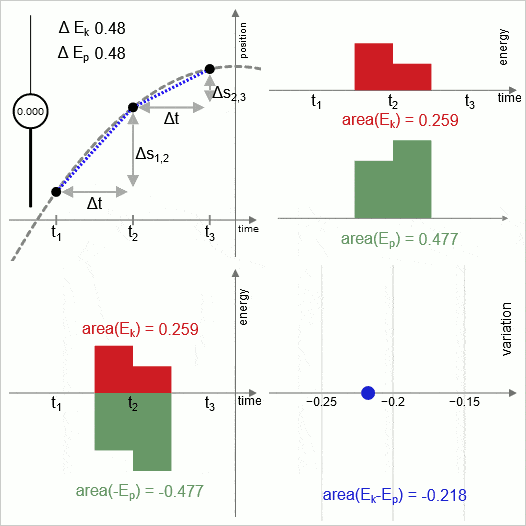Diagram 4 - Evaluation of area

Diagram 4 demonstrates a property that we get for free. We get it for free in the sense that it doesn't require any additional assumption; it follows logically.

The two time intervals ##t_{1,2}## and ##t_{2,3}## are displayed in three of the four subpanels: upper-left, upper-right, and lower-left. The time intervals are set up to be of equal duration.

In the upper-right sub-panel: the height of each bar represents the value of the energy corresponding to that time interval. The number displayed in red is the summed area of the two red bars; the number displayed in green is the the summed area of the two green bars.

Reminder: the energies that are displayed in this diagram are hypothetical energies. The variation sweep is hypothetical: at each position of the movable point the diagram shows what the energies would be if the object would move along that particular trial trajectory.

Stating the two different kinds of change explicity:
- Rate of change of actual energy as an object is moving along the true trajectory.
- Rate of change of hypothetical energy (as a function of variation sweep)

In motion along the true trajectory one form of energy is transformed into the other; the energies are counter-changing; ##\Delta E_k = -\Delta E_p##

Diagram 4 illustrates that in sweeping out variation the (hypothetical) energies are co-changing.

In the case of variation sweep: obviously the only way to evaluate the response to variation sweep is by taking the derivative with respect to the position coordinate. In the case of the motion along the true trajectory: as expressed with eq. (1.13): If you express the physics taking place in terms of energy you gain the option of taking the derivative with respect to the position coordinate. This is what allows us to connect the evaluation of the hypothetical variation sweep to the actual energies.

There is precisely one point where the hypothetical rate of change and the physical rate of change have the same magnitude: at the sweet spot. Variational calculus capitalizes on that correspondence. Variational calculus uses the response to variation to obtain information about the motion along the true trajectory.

What we are looking to do is comparison of the rate of change of kinetic energy to the rate of change of potential energy. In the hypothetical variation sweep the energies are co-changing, so in order to compare them we need to subtract one from the other. (By convention it is the potential energy that gets the minus sign.)

Given that we are subtracting the potential energy from the kinetic energy: in the lower-left subpanel the green area is displayed as area below the coordinate's zero line. We can treat the green area as signed area; area is below the zero point of the coordinate system is counted as negative.

The lower-right sub-panel represents in blue the result of the subtraction.

The motion of the blue dot over the diagram represents how the value 'area(##E_k-E_p##)' is responding to variation sweep.

At the point where the variation hits the sweet spot the value of 'area(##E_k-E_p##)' is stationary. That means: at the point where the variation hits the sweet spot the rate of change of summed red area matches the rate of change of summed green area. This is of course not a coincidence.

The two time intervals ##t_{1,2}## and ##t_{2,3}## are set to be equal in duration. Hence:

The rate of change of green area:
At the sweet spot the rate at which the summed green area changes is equal to the rate of change of potential energy as a function of sweeping out variation.

The rate of change of red area:
At the sweet spot the difference in rate of change of the left and right red bar is such that the rate of change of summed red area is equal to the rate of change of kinetic energy as a function of sweeping out variation.

Summerizing:
There is a point in the variation sweep where the rate of change of kinetic energy matches the rate of change of potential energy. If the time intervals are set to be of equal duration then it follows mathematically that the rate of change of summed red area matches the rate of change of summed green area.

Integral
The logic that is demonstrated in diagram 4 is valid at any scale, down to infinitisimal.

When concatenating units of operation along the length of the overall time the bars end up exactly adjacent; no overlap.

This explains why the *integral* of the quantity (##E_k - E_p##) has the property that it is stationary when the variation hits the sweet spot. The value of (##E_k - E_p##) already has that property at the infinitisimal scale, and from there it propagates to the value of the integral of (##E_k - E_p##), as a function of variation sweep.

The crucial point: the above reasoning does not require making any additional physical assumption. Hence: in any situation where the Work-Energy theorem holds good it follows that Hamilton's stationary action will hold good.

For the sake of completeness I give an outline for how to derive the Euler-Lagrange equation using the unit of operation demonstrated in diagram 1

I learned this approach to the derivation from an article by Preetum Nakkiran, titled Geometric derivation of Euler-Lagrange equation.

In the following the notation conventions for mechanics are used:
t for 'time'
s for 'position'
v for 'velocity'
a for 'acceleration'

##S## for Hamilton's action
##S_k## for the kinetic energy component of Hamilton's action
##S_p## for the potential energy component of Hamilton's action

The small variation of position coordinate will be represented with a factor ##\delta s##. The potential energy and the kinetic energy each respond to that variation, responding differently.

Recapitulating:
-as an object is in motion along the true trajectory the kinetic energy and potential energy are counter-changing.
-When you are sweeping out variation: the kinetic energy and potential energy are co-changing

The following derivation is for the hypothetical variation, therefore the potential energy must be entered into the derivation as minus potential energy.

The response of the potential energy component of Hamilton's action:
$$\delta S_p = -\frac{dE_p}{ds} \delta s \qquad (2.1)$$
The response of the kinetic energy component of Hamilton's action:
On both sides of ##t_2## the velocity changes; one side increases, the other side decreases.</p>

Before the change:
$$v_{1,2} = \frac{\Delta s_{1,2}}{\Delta t} \qquad v_{2,3} = \frac{\Delta s_{2,3}}{\Delta t} \qquad (2.2)$$
$$v_{1,2} = \frac{\Delta s_{1,2 } + \delta s}{\Delta t} \qquad v_{2,3} = \frac{\Delta s_{2,3} - \delta s}{\Delta t} \qquad (2.3)$$
The changes of the velocities:
$$\Delta v_{1,2} = \frac{\delta s}{\Delta t} \qquad \Delta v_{2,3} = \frac{- \delta s}{\Delta t} \qquad (2.4)$$
The change of the kinetic energy component of Hamilton's action:
$$\delta S_k = \frac{dE_{k(1,2)}}{dv_{1,2}}\Delta v_{1,2} + \frac{dE_{k(2,3)}}{dv_{2,3}}\Delta v_{2,3} \qquad (2.5)$$
$$\delta S_k = - \left(\frac{dE_{k(2,3)}}{dv_{2,3}} - \frac{dE_{k(1,2)}}{dv_{1,2}} \right) \frac{\delta s}{\Delta t} \qquad (2.6)$$
The total time is divided in equal length sub-intervals, so the move from interval (1,2) to the adjacent (2,3) can be rearranged to the form of taking a time derivative:
$$\frac{dE_{k(2,3)}}{dv_{2,3}} - \frac{dE_{k(1,2)}}{dv_{1,2}} = \frac{d}{dt}\frac{dE_{k(1,2)}}{dv_{1,2}}\Delta t \qquad (2.7)$$
Combining (2.6) and (2.7) we obtain an expression for the change of the kinetic energy component of Hamilton's action as a function of &delta;<i>s</i>:
$$\delta S_k = -\frac{d}{dt} \frac{dE_{k(1,2)}}{dv_{1,2}} \delta s \qquad (2.8)$$
Combining the two responses:
$$\delta S = \delta S_p + \delta S_k = \left( -\frac{d E_p}{ds} - \frac{d}{dt} \frac{dE_{k(1,2)}}{dv_{1,2}} \right) \delta s \qquad (2.9)$$
The variation ##\delta s##, having served its purpose, can now be eliminated. The stationary-in-response-to-variation condition is satisfied when the following is satisfied:
$$-\frac{d E_p}{ds} - \frac{d}{dt} \frac{dE_k}{dv} = 0 \qquad (2.10)$$
Flipping the two minus signs does not change the equation:
$$\frac{d E_p}{ds} + \frac{d}{dt} \frac{dE_k}{dv} = 0 \qquad (2.11)$$
The Euler-Lagrange equation with the Lagrangian denoted with the symbol '##L##':
$$\frac{\partial L}{\partial s} - \frac{d}{dt} \frac{\partial L}{\partial v} = 0 \qquad (2.12)$$
Substituting the Lagrangian (##E_k - E_p##) into that:
$$\frac{\partial (E_k - E_p)}{\partial s} - \frac{d}{dt} \frac{\partial (E_k - E_p)}{\partial v} = 0 \qquad (2.13)$$

(2.13) and (2.11) are the same equation.

Narrowing down to what is necessary

The Euler-Lagrange equation is very compact. The Euler-Lagrange equation is an equation that has been narrowed down to what is necessary. You don't need more; the Euler-Lagrange equation is sufficient.

In other words: in retrospect we can see that all elements that were removed during the process of deriving the Euler-Lagrange equation were unnecessary elements.

The standing convention is to state the functional as an integral, but at the end the integral is no longer there; the Euler-Lagrange equation is a differential equation.

The unit of operation of diagram 1 explains why the Euler-Lagrange equation is a differential equation: the unit of operation is valid at infinitisimal scale. The logic operates at the infinitisimal scale, and from there it propagates out to the trajectory as a whole.

The integral that is present at the start is redundant, and is accordingly removed in the process of deriving the Euler-Lagrange equation

The relation between variational calculus and differential calculus

To make the comparison I take a simple case that is a natural case for application of variational calculus: the catenary.

As we know, mathematically the curve of a hanging chain is described by the hyperbolic cosine; the hyporbolic cosine gives the height as a function of the horizontal coordinate.

We have with the catenary that the force that is acting is perpendicular to the horizontal. And if the length of the catenary is changed then the change in the curve is change perpendicular to the horizontal.

Variational calculus pivots the direction of the differentiation. Variational calculus is a form of differential calculus where instead of differentiating with respect to ##x## (the horizontal coordinate), the differentiation is with repect to ##y##, in such a way that you do end up with a function that gives the height of the catenary as a function of the horizontal coordinate.

The meaning of the 'stationary' criterium

I have noticed that authors of physics textbooks tend to have a cavalier attitude towards the question: "What is the meaning of the 'stationary' criterium?" The authors will acknowledge that the name 'stationary action' is better, but will then express that to actually use that name would be pedantic.

The purpose of the following discussion is to show that to understand the meaning of the 'stationary' criterium is key.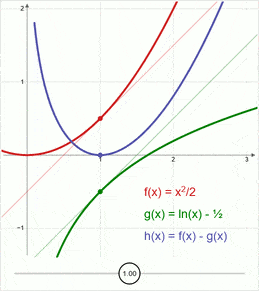Diagram 5 - The blue curve has a point where it is stationary

In Diagram 5 the red curve and the green curve are both ascending functions.
Red: ##f(x) = \frac{1}{2} x^2##
Green: ## g(x) = ln(x) ##

Problem: at what x-coordinate do the functions ##f(x)## and ##g(x)## have the same slope?
Phrased differently: at what x-coordinate is the rate of change of ##f(x)## equal to the rate of change of ##g(x)##?

The direct way to answer that question is to start with taking the derivative of each function, and then solve for the point where the two derivatives have the same value:
$$\frac{f(x)}{dx} = \frac{g(x)}{dx} \qquad (3.1)$$
$$\frac{\tfrac{1}{2}x^2}{dx} = \frac{ln(x)}{dx} \qquad (3.2)$$
$$x = \frac{1}{x} \qquad (3.3)$$
There is also a more convoluted way to address the problem:
I will refer to this more convoluted way as constructing a 'mathematical action':
Create a third function by subtracting the second function from the first function.
$$h(x) = f(x) - g(x) \qquad (3.4)$$
$$h(x) = \tfrac{1}{2} x^2 - ln(x) \qquad (3.5)$$
In diagram 5 the blue curve represents ##h(x)##, the 'mathematical action'.

In diagram 4 the motion of the blue dot in response to variation sweep traces a curve, and that curve has a stationary point. That stationary point is the point where the rate of change of kinetic energy matches the rate of change of potential energy.

Last edited:
•PeroK